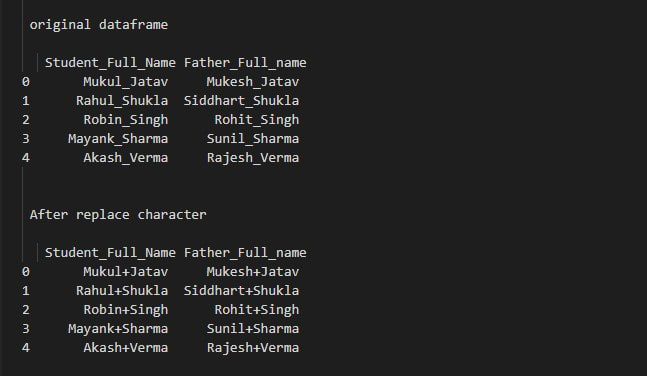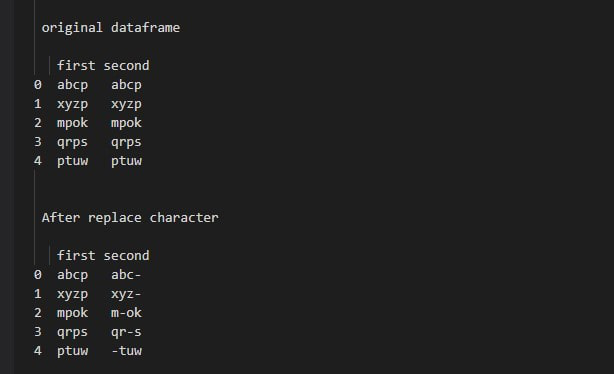# Replace Characters in Strings in Pandas DataFrame

In this article, we are going to see how to replace characters in strings in pandas dataframe using Python.

We can replace characters using str.replace() method is basically replacing an existing string or character in a string with a new one. we can replace characters in strings is for the entire dataframe as well as for a particular column.

Syntax: str.replace (old_string, new_string, n=-1, case=None, regex=True)

Parameters:

• old_string: string to be replaced.
• new_string: string or callable to replace instead of pat.
• n: Number of replacement to make in a single string, default is -1 which means All.
• case: Takes boolean value to decide case sensitivity. Make false for case insensitivity.
• regex: Boolean value, if True assume that the passed pattern is a regex.

Return Type: return a copy of the object with all matching occurrences of old_string replaced by new_string.

Example 1: The following program is to replace a character in strings for the entire dataframe.

## Python3

 `# import pandas` `import` `pandas as pd`   `data ``=` `{``'Student_Full_Name'``:  [``'Mukul_Jatav'``, ``'Rahul_Shukla'``,` `                               ``'Robin_Singh'``, ``'Mayank_Sharma'``,` `                               ``'Akash_Verma'``],` `        ``'Father_Full_name'``: [``'Mukesh_Jatav'``, ``'Siddhart_Shukla'``,` `                             ``'Rohit_Singh'``, ``'Sunil_Sharma'``,` `                             ``'Rajesh_Verma'``]` `        ``}` `# create an dataframe` `df ``=` `pd.DataFrame(data, columns``=``[``'Student_Full_Name'``, ` `                                 ``'Father_Full_name'``])`   `# print dataframe` `print``(``" original dataframe \n"``, df)`   `# replace '_' with '-'` `df ``=` `df.replace(``'_'``, ``'+'``, regex``=``True``)`   `# print dataframe` `print``(``" After replace character \n"``, df)`

OutputExample 2: The following program is to replace a character in strings for a specific column.

## Python3

 `# import pandas` `import` `pandas as pd`   `data ``=` `{``'first'``:  [``'abcp'``, ``'xyzp'``, ``'mpok'``, ` `                   ``'qrps'``, ``'ptuw'``],` `        ``'second'``: [``'abcp'``, ``'xyzp'``, ``'mpok'``, ` `                   ``'qrps'``, ``'ptuw'``]` `        ``}` `# create an dataframe` `df ``=` `pd.DataFrame(data, columns``=``[``'first'``, ``'second'``])`   `# print dataframe` `print``(``"\n original dataframe \n\n"``, df)`   `# replace '_' with '='` `df[``'first'``] ``=` `df[``'first'``].``str``.replace(``'p'``, ``'-'``)`   `# print dataframe` `print``(``"\n\n After replace character \n\n"``, df)`

Output:Another approach to replace characters in strings in a Pandas DataFrame without using the replace method is to use a combination of the apply and lambda functions.

The apply function allows you to apply a specific function to a certain axis of the DataFrame. You can use the lambda function as the input to apply, which allows you to define a function inline. In this case, you can use the lambda function to iterate over each element in the column, and use string manipulation techniques to replace the desired characters.

Here is an example of how you could use this approach to replace the _ character with a + character in the Student_Full_Name column of the DataFrame from the previous example:

`df['Student_Full_Name'] = df['Student_Full_Name'].apply(lambda x: x.replace('_', '+'))`

Note that this approach may not be as efficient as using the replace method, as it requires the creation of a new function for each element in the column. However, it can be useful in cases where the replace method is not suitable or when you need to perform more complex string manipulation operations.

## Python3

 `import` `pandas as pd`   `def` `replace_char(s):` `    ``return` `s.replace(``'_'``, ``'+'``)`   `data ``=` `{``'Student_Full_Name'``:  [``'Mukul_Jatav'``, ``'Rahul_Shukla'``,` `                               ``'Robin_Singh'``, ``'Mayank_Sharma'``,` `                               ``'Akash_Verma'``],` `        ``'Father_Full_name'``: [``'Mukesh_Jatav'``, ``'Siddhart_Shukla'``,` `                             ``'Rohit_Singh'``, ``'Sunil_Sharma'``,` `                             ``'Rajesh_Verma'``]` `        ``}` `df ``=` `pd.DataFrame(data, columns``=``[``'Student_Full_Name'``, ``'Father_Full_name'``])` `df[``'Student_Full_Name'``] ``=` `df[``'Student_Full_Name'``].``apply``(``lambda` `x: x.replace(``'_'``, ``'+'``))`   `print``(df)`

Output:

```  Student_Full_Name Father_Full_name
0       Mukul+Jatav     Mukesh_Jatav
1      Rahul+Shukla  Siddhart_Shukla
2       Robin+Singh      Rohit_Singh
3     Mayank+Sharma     Sunil_Sharma
4       Akash+Verma     Rajesh_Verma```

Whether you're preparing for your first job interview or aiming to upskill in this ever-evolving tech landscape, GeeksforGeeks Courses are your key to success. We provide top-quality content at affordable prices, all geared towards accelerating your growth in a time-bound manner. Join the millions we've already empowered, and we're here to do the same for you. Don't miss out - check it out now!

Previous
Next